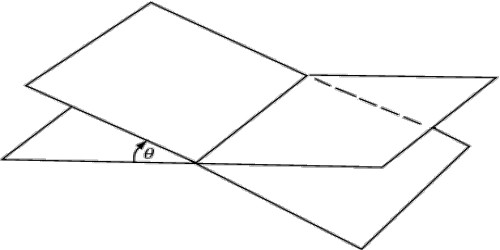### Geometrical Property of Altitudes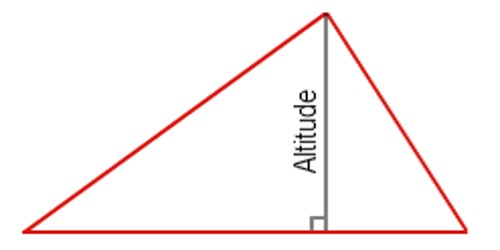Geometrical Property of Altitudes Sometimes the opposite side isn’t quite long enough to draw an altitude, so we are allowed to extend it to make an altitude possible. This line containing the opposite side is called the extended base of the altitude. The intersection of the extended base and t.....

### Altitude of a TriangleAltitude of a Triangle In geometry, an altitude of a triangle is a line segment through a vertex and perpendicular to a line containing the base. The definition of the altitude of a triangle is a line that extends from one vertex of a triangle perpendicular to the opposite side. Sometimes the opp.....

### Properties of Angles of a Triangle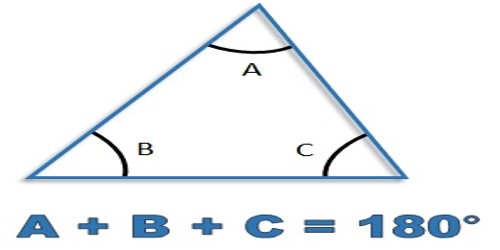Triangle is a plane figure with three straight sides and three angles. The three angles always add to 180°. The vertex is a corner of the triangle. Every triangle has three vertices. The interior angles of a triangle always add up to 180°. The exterior angles of a triangle always add up to 360�.....

### Order of a Surd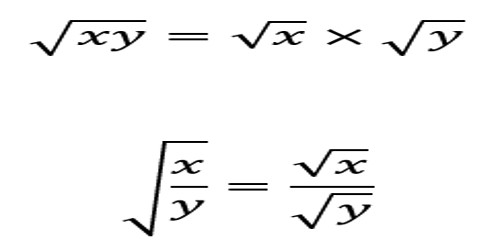Order of a Surd Definitions of surds: A root of a positive real quantity is called a surd if its value cannot be exactly determined. It is a number that can’t be simplified to remove a square root (or cube root etc). For example, each of the quantities √3, ∛7, ∜19, (16)^2/5 etc. is a [&he.....

### Induction Proof – Problems with Solutions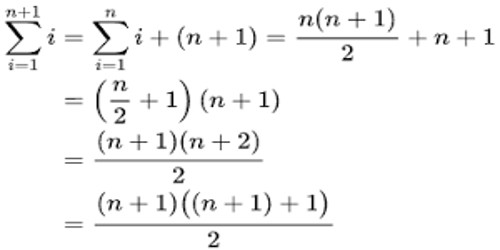Induction Proof – Problems with Solutions Mathematical induction is a technique for proving a statement – a theorem, or a formula — that is asserted about every natural number. It is a mathematical proof technique used to prove a given statement about any well-ordered set. Most commonly.....

### Theorem of Three Perpendiculars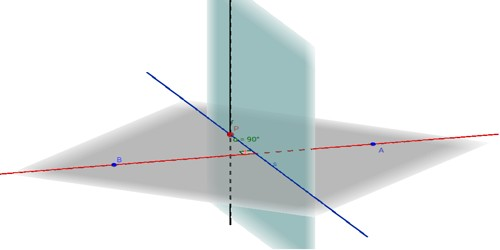Theorem of Three Perpendiculars A line is said to be perpendicular to another line if the two lines intersect at a right angle. Explicitly, a first line is perpendicular to a second line if (1) the two lines meet, and (2) at the point of intersection the straight angle on one side of the first [&.....

### Converse of the Theorem on Parallel Lines and Plane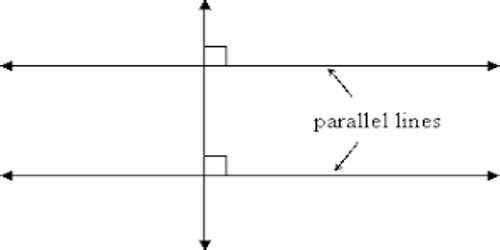Converse of the theorem on parallel lines and plane: Theorem: If two straight lines are parallel and if one of them is perpendicular to a plane, then the other is also perpendicular to the same plane. When lines and planes are perpendicular and parallel, they have some interesting properties. Lin.....

### Theorem on Parallel Lines and PlaneTheorem on Parallel Lines and Plane Theorem: If two straight lines are parallel and if one of them is perpendicular to a plane, then the other is also perpendicular to the same plane. When lines and planes are perpendicular and parallel, they have some interesting properties. Lines and planes are.....

### Theorem on Co-planar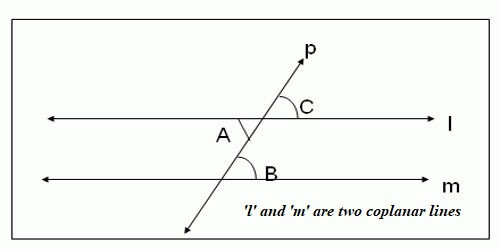Theorem on Co-planar A set of points, lines, line segments, rays or any other geometrical shapes that lie on the same plane are said to be Coplanar. In geometry, a set of points in space are coplanar if there exists a geometric plane that contains them all. For example, three points are always co.....

### Theorems on Straight Lines and Plane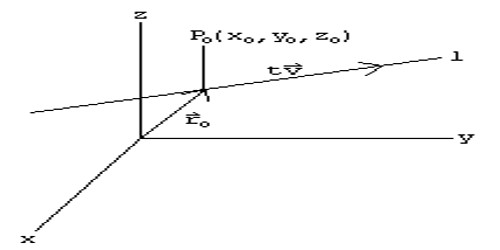Theorems on Straight Lines and Plane In mathematics, the term “line” actually refers to the “straight line”. It is a one-dimensional figure that extends in two opposite directions. A straight line can be defined as an interconnected set of an infinite number of points. The length of a lin.....

### Axioms: Solid Geometry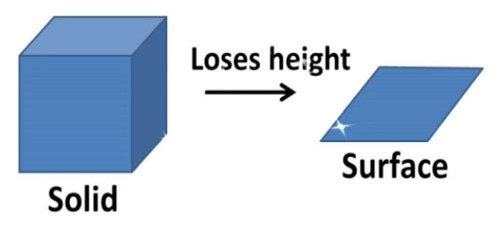Axioms: Solid Geometry Axioms involving reflection at a plane were approached through physical observations of reflection from a plane mirror. Axioms are general statements made about real numbers. Sometimes they are called algebraic postulates. Often what they say about real numbers holds true f.....